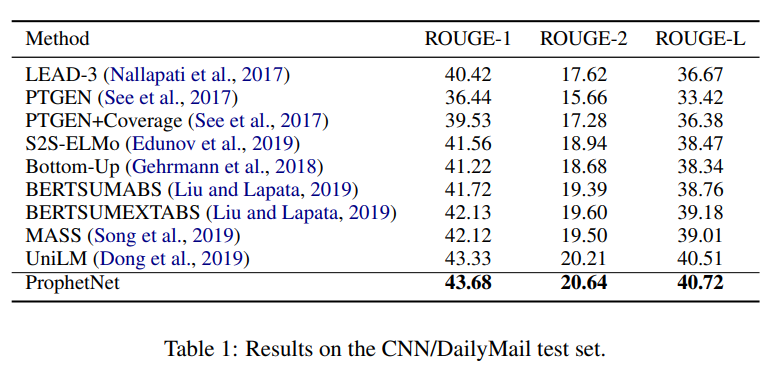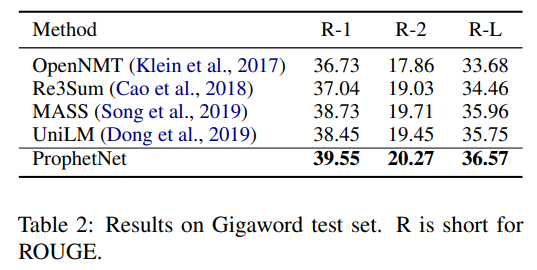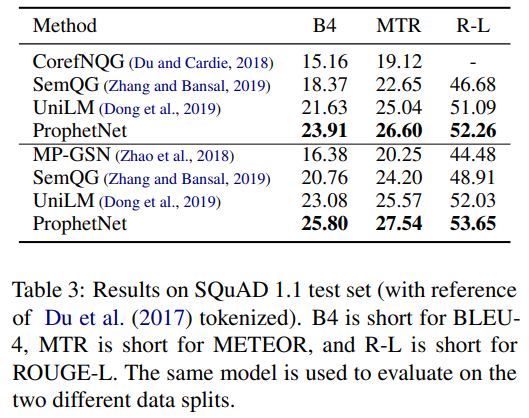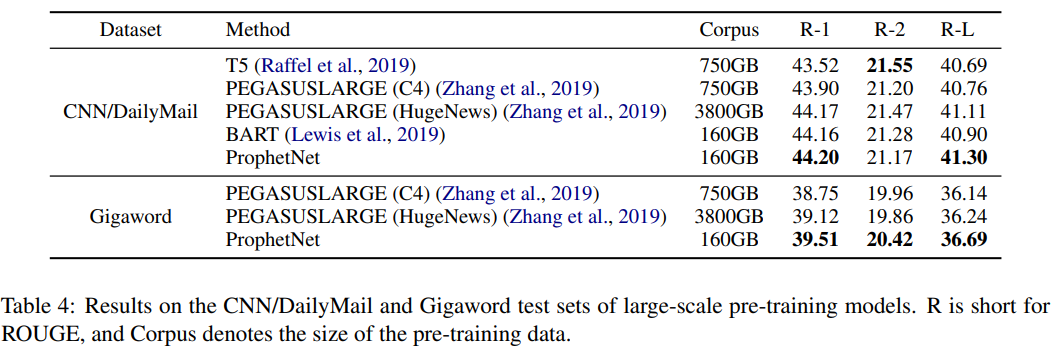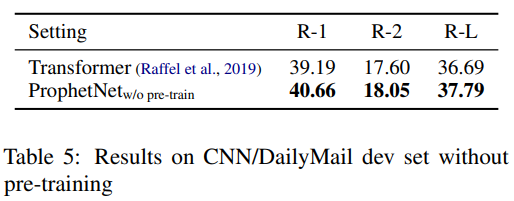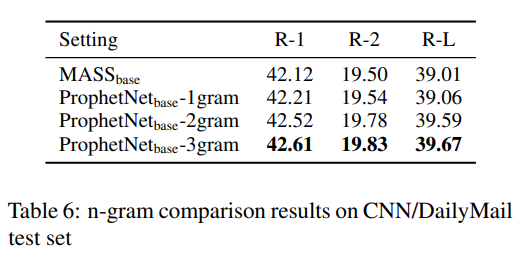《ProphetNet: Predicting Future N-gram for Sequence-to-Sequence Pre-training》（ProphetNet，2020，EMNLP Findings），partially referred to Fairseq-0.9.0 and MASS

microsoft/ProphetNet: Code for EMNLP20 paper: "ProphetNet: Predicting Future N-gram for Sequence-to-Sequence Pre-training" (github.com)

transformers也有相应实现

## 摘要

• 一个新的自监督训练目标：future n-gram prediction以及n-stream自监督机制
• 分别基于16G和160G的语料库，预训练ProphetNet，在CNN/DailyMail、Gigaword、SQuAD1.1上测试摘要和问题生成任务，ProphetNet达到SOTA（compared to the models using the same scale pre-training corpus.）

## 介绍

• AR：给定文本$$x=(x_1,...,x_T)$$，将似然分解为$$p(x)=\prod_{t=1}^Tp(x_t|x_{<t})$$，训练方式为teacher forcing，

optimized to predict the next token given all previous context tokens at each time step

• 倾向于关注最近的token，因为local correlation如n-gram的关系通常比长期依赖更强，并且相应的teacher forcing关注one-step-prediction，对更远的token没有准确的偏置（bias）
• 因此AR的语言模型存在“偏”的问题——overfit local token combination，underfit global coherence，这在decode种使用greedy search时更为明显
• ProphetNet：

• 自监督目标：future n-gram prediction
• 两个目的：
• 训练阶段，模型应当能在一个time step同时预测future n-gram
• 在fine-tune和推理阶段，模型能够被转换为只预测下一个token（和原始的seq2seq类似）
• 将xlnet的two-stream自监督，扩展为n-steam自监督
• 一个main stream自监督，和原始的Transformer的自监督机制相同
• n个额外的自监督stream，分别用于n-gram prediction中的n个token。即，预训练时第i个预测stream关注main stream里的hidden states，以预测第i个future token
• 而main stream被所有的预测steam共享，因此推理时可以关闭预测stream，每个time step只预测接下来的一个token

• 16G的语料和Bert的语料相同，160G的语料和BART的语料相同——后者达到SOTA，并且只用了1/3的BART预训练epoch，以及1/5的T5（PEGASUS）语料

## ProphetNet

• 基于Transformer结构

### seq2seq learning

• 给定文本对$$(x,y)=(x_1,...,x_M,y_1,...,y_T)$$
• 建模条件似然$$p(y|x)=\prod_{t=1}^Tp(y_t|y_{<t},x)$$——encoder编码x，decoder建模条件似然

### Future N-gram Prediction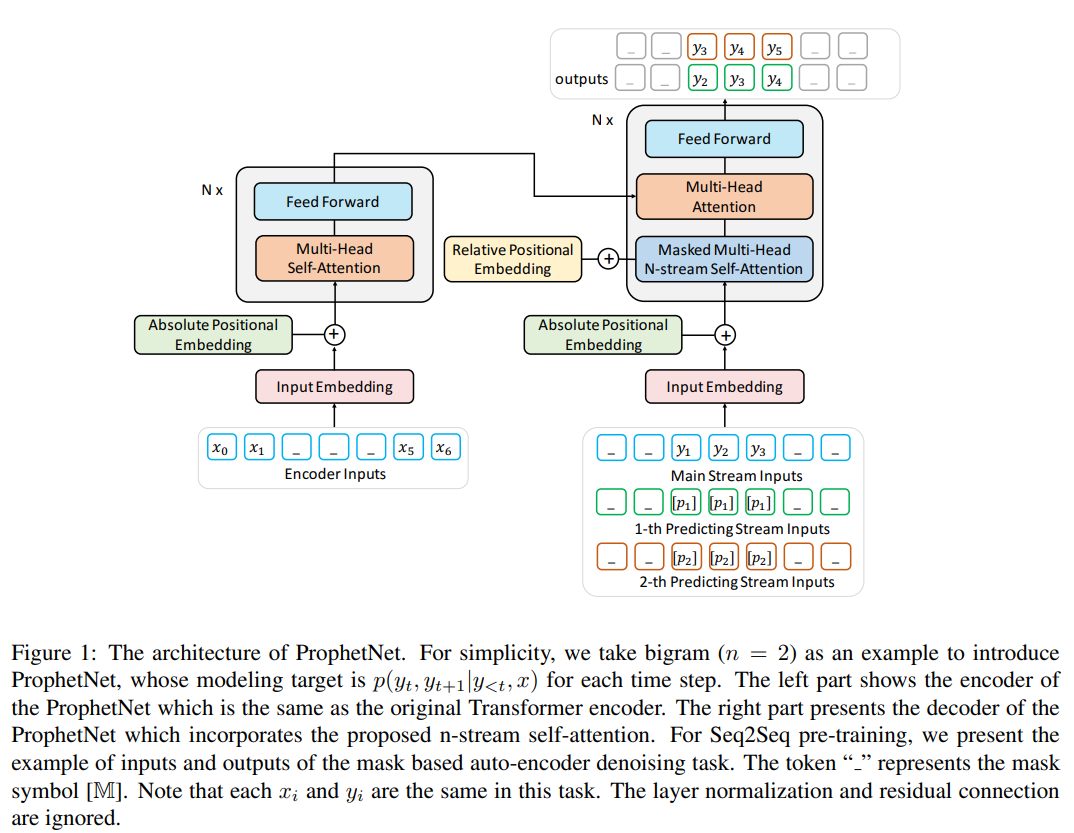• 将条件似然中$$p(y_t|y_{<t},x)$$改为：$$p(y_{t:t+n-1}|y_{<t},x)$$

• 给定x，ProphetNet将x编码（和原始的Transformer encoder一样）：$$H_{enc}=Encoder(x_1,...,x_M)$$$$H_{enc}$$为句子表征

• decoder在每个time step输出n个概率，即$$p(y_t|y_{<t},x),...,p(y_{t+n-1}|y_{<t},x)=Decoder(y_{<t},H_{enc})$$

• future n-gram prediction目标可以形式化为：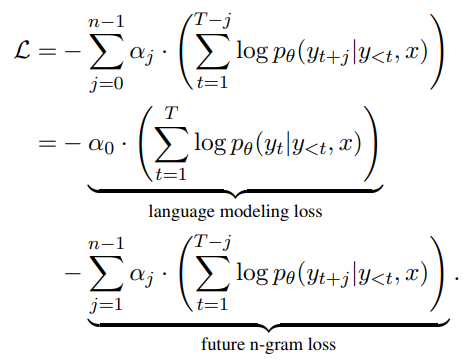• 分为两个部分：
• 条件的LM损失（同原始损失的一致）
• n-1个future token的预测损失，其中$$\alpha_j$$用于平衡权重，本文设置$$\alpha_j=\frac{\gamma^j}{\sum_{i=0}^{n-1}\gamma^i}$$，其中$$\gamma$$为衰减系数（attenuation coefficient）

### N-Stream Self-Attention

• 除了原始transformer decoder中的掩码多头自注意力（main stream），还加入了n-stream自注意力机制，第i个预测流负责建模概率$$p(y_{t+i-1}|y_{<t},x)$$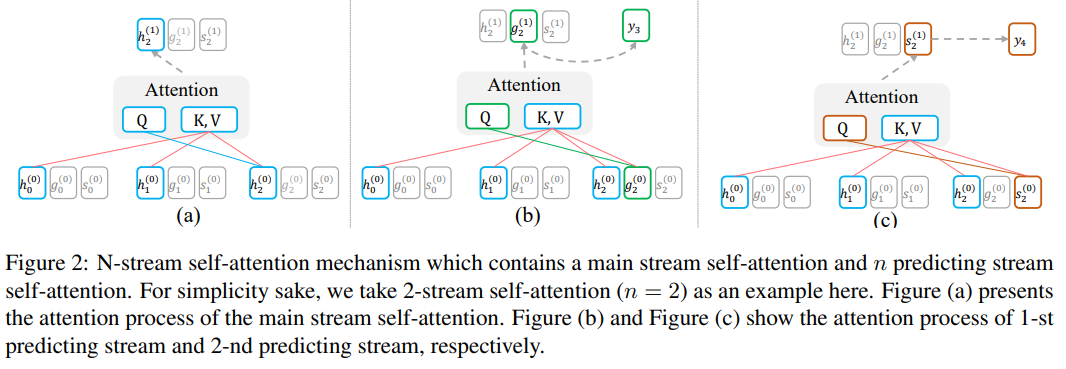• 上图h stream为main stream，g stream和s steam分别用于预测第一个、第二个token

• main stream和传统的transformer decoder的掩码多头自注意力相同，使用一个三角矩阵控制每个位置只能注意前面的token：$$H^{(k+1)}=MultiHead(H^{(k)},H^{(k)},H^{(k)})$$，其中$$H^{(k)}=(h_0^{(k)},...,h_T^{(k)})$$表示main stream中第k个层的隐藏状态

• 上图b，显示1-st预测流和其隐藏状态可以计算为：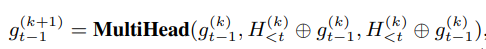• 其中，$$g_{t-1}^{(k+1)}$$表示时间步t-1时，1-st预测流的第k+1层的隐藏状态
• $$g_{t-1}^{(k)}$$作为注意力的query，而value和key为main stream的当前时间步前的隐藏状态
• 为了使$$g_{t-1}^{(k+1)}$$position-aware，还取$$g_{t-1}^{(k)}$$作为注意力的value和key
• 类似地，2-nd预测流为：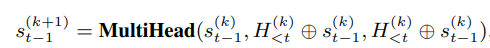• 二者的初始化token、绝对位置的嵌入、相对位置的计算是不同的（预测流通过特殊的token初始化，而非previous tokens）

• 在训练中，共享每个预测流和main stream的参数，因此可以简单地将ProphetNet decoder转化为transformer decoder

• 为了增强decoder的位置信息，将绝对位置嵌入和T5提出的bucket relative positional calculation结合起来

• ProphetNet要在每个时间步内恢复masked token span中的future n tokens（MASS每个时间步只会恢复下一个token）

## 实验和结果

### 预训练

• 模型参数：
• 12层encoder+12层decoder
• embedding size（hidden size）：1024
• feed forward size：4096
• batch size：1024，steps：500K
• 设置n为2
• 预训练数据集：
• 同BERT：BookCorpus+English Wikipedia
• 类似BART，但BART的数据集CC-News未公开，使用 similar public news corpus REALNEWS
• 预训练设置：
• 输入长度为512，随机覆盖
• 衰减系数为1.0
• 屏蔽长度为token总数的15%

### 微调

CNN/DailyMail、Gigaword——summarization

### 结果